Name:    Chapter 10 Test

Multiple Choice
Identify the choice that best completes the statement or answers the question.

1.

There are 12 sixth graders, 14 seventh graders, and 7 eighth graders in a gym class. The gym teacher randomly selects one student to collect balls. In how many ways can choosing not a seventh grader occur?
 a. 33 ways c. 19 ways b. 21 ways d. 14 ways

Use the spinner to determine the probability of the event.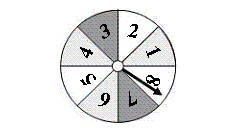2.

Not spinning a 4
 a.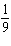c.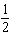b.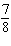d.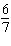3.

Spinning a number less than 10
 a.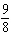c. 1 b.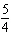d.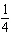You roll a number cube. Determine the theoretical probability of the event.

4.

Rolling a 3
 a.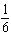c.b.d.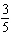5.

Rolling a multiple of  2
 a.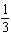c.b.d. 1

The bar graph shows the results of spinning the spinner 40 times. Find the experimental probability of the event.

6.

Spinning a 4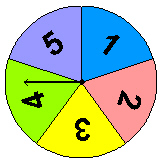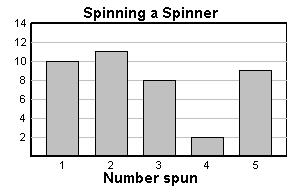a.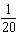c.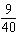b.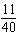d.7.

Spinning a number greater than 1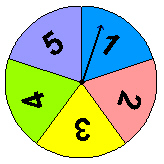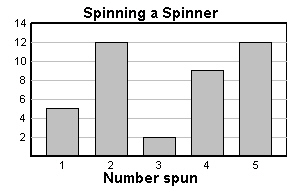a.c.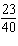b.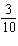d.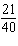Use the Fundamental Counting Principle to find the total number of possible outcomes.

8.

 MP3 Player Memory 1 GB, 2 GB, 4 GB , 8 GB, 16 GB, 32 GB Color Purple, Black, White
 a. 18 c. 19 b. 9 d. 14

You randomly choose one of the chips. Without replacing the first chip, you choose a second chip. Find the probability of choosing the first chip, then the second chip.

9.

Green and White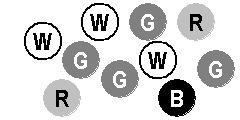a.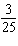c.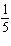b.d.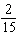10.

White and not a Black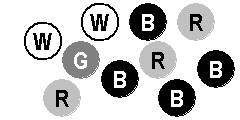a.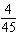c.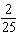b.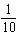d.You randomly choose one of the tiles shown below. Find the favorable outcomes of the event.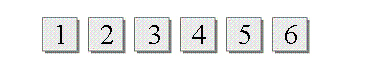1.

Choosing an odd number

2.

Choosing a number divisible by 3

3.

You want to estimate the number of students who buy school lunch. You survey students as they wait in line at the school cafeteria. Determine whether the sample is biased or unbiased. Explain.

4.

The tables show the points scored by two school basketball teams during a season.
 Team A 65 43 54 55 49 68 48 64 51 44 45 46 60 63 44 52 53 57 36 60 31

 Team B 77 67 86 87 63 89 68 86 90 76 67 90 75 83 62 64 75 89 69 60 72
a.   Compare the populations using measures of center and variation.
b.   Express the difference in the measures of center as a multiple of each measure of variation.